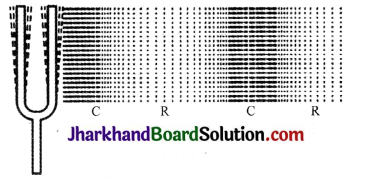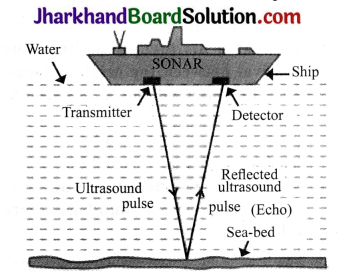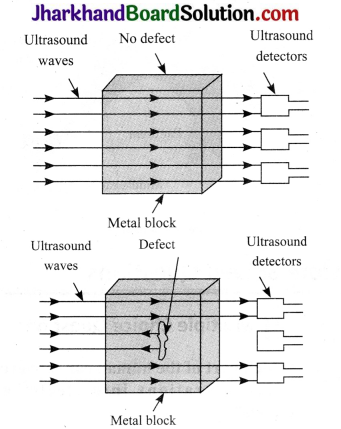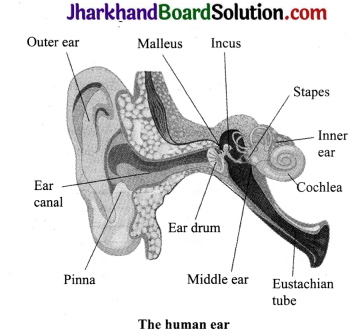# JAC Class 9th Science Solutions Chapter 12 Sound

## JAC Board Class 9th Science Solutions Chapter 12 Sound

JAC Class 9th Science Sound InText Questions and Answers

Poge 162

Question 1.
How does the sound produced by a vibrating object in a medium reach your ear?
When an object vibrates, it sets the particles of the medium around it in vibration. The particles in the medium in contact with the vibrating object are displaced from their equilibrium position. It then exerts a force on the adjacent particles. After displacing the adjacent particle, the first particle of the medium comes back to its original position. This process continues in the medium till the sound reaches our ear.

Page 163

Question 1.
Explain how sound is produced by your school bell.
When the bell continues to vibrate forward and backward, it creates a series of compressions and rarefactions resulting in the production of sound.

Question 2.
Why are sound waves called mechanical waves?
Sound waves need material medium to propagate, therefore, they are called mechanical waves. Sound waves can propagate through a medium only because of the interaction of the particles present in that medium.Question 3.
Suppose you and your friend are on the moon. Will you be able to hear any sound produced by your friend?
No, because a sound wave needs a medium through which it can propagate. Since there is no atmosphere on the moon, no material medium is available and we cannot hear any sound on the moon.

Page 166

Question 1.
Which wave property determines:
(a) loudness
(b) pitch?
(a) Amplitude
(b) Frequency

Question 2.
Guess which sound has a higher pitch: guitar or car horn?
Guitar has a higher pitch than car horn, because sound produced by the strings of guitar has a higher frequency than that of a car horn. The higher the frequency, the higher is the pitch.

Page 166

Question 1.
What are wavelength, frequency, time period and amplitude of a sound wave?

1. Wavelength: The distance between two consecutive compressions or two consecutive rarefactions is known as wavelength. Its SI unit is metre (m).
2. Frequency: The number of complete oscillations per second is known as the frequency of a sound wave. It is measured in hertz (Hz).
3. Time period: The time taken by two consecutive compressions or rarefactions to cross a fixed point is called the time period of the wave.
4. Amplitude: The maximum height reached by the crest or the maximum depth reached by the trough of a
sound wave is called its amplitude.

Question 2.
How are the wavelength and frequency of a sound wave related to its speed?
Speed, wavelength and frequency of a sound wave are related by the following equation:
Speed (v) = Wavelength (λ) x Frequency (v)
V = λ × v

Question 3.
Calculate the wavelength of a sound wave whose frequency is 220 Hz and speed is 440 m/s in a given medium.
Frequency of the sound wave, v = 220 Hz
Speed of the sound wave, v = 440 ms-1
For a sound wave,
Speed = Wavelength × Frequency
v = λ × v
∴ λ = $$\frac{v}{v}$$ = $$\frac{440}{220}$$ = 2
Hence, the wavelength of the sound wave is 2 m.

Question 4.
A person is listening to a tone of 500 Hz sitting at a distance of 450 m from the source of the sound. What is the time interval between successive compressions from the source?
The time interval between two successive compressions is equal to the time period of the wave. This time period is reciprocal of the frequency of the wave and is given by the relation:
T = $$\frac{1}{\text { Frequency }}$$ = $$\frac{1}{500}$$ = 0.002 s

Page 166

Question 1.
Distinguish between loudness and intensity of sound.
Intensity of a sound wave is defined as the amount of sound energy passing through a unit area per second. Loudness is a measure of the response of the ear to the sound. The loudness of a sound is defined by its amplitude.

Page 167

Question 1.
In which of the three media, air, water or iron, does sound travel the fastest at a particular temperature?
The speed of sound depends on the nature of the medium. Sound travels the fastest in solids. Its speed decreases in liquids and it is the slowest in gases. Therefore, for a given temperature, sound travels fastest in iron.

Page 168

Question 1.
An echo is heard in 3s. What is the distance of the reflecting surface from the source, given that the speed of sound is 342 ms-1?
Speed of sound, v = 342 ms-1
Echo returns in time, t = 3s
Distance travelled by sound
= v × t = 342 × 3 = 1026 m
In the given time interval, sound has to travel a distance that is twice the distance between the reflecting surface and the source.
Hence, the distance of the reflecting surface from the source
= $$\frac{1026}{2}$$ m = 513 m.

Page 169

Question 1.
Why are the ceilings of concert halls curved?
The ceilings of concert halls are curved so that the sound, after reflection (from the walls), spreads uniformly in all directions.

Page 170

Question 1.
What is the audible range of the average human ear?
The audible range of an average human ear is 20 Hz to 20,000 Hz.

Question 2.
What is the range of frequencies associated with
(a) Infrasound?
(b) Ultrasound?
(a) Infrasound has frequencies less than 20 Hz.
(b) Ultrasound has frequencies more than 20,000 Hz.

Page 172

Question 1.
A submarine emits a sonar pulse, which returns from an underwater cliff in 1.02s. If the speed of sound in salt water is 1531 m/s, how far away is the cliff?
Time taken by the sonar pulse to return, t = 1.02s
Speed of sound in salt water, v = 1531 ms-1
Distance travelled by the sonar pulse
= Speed of sound × Time taken
= 1.02 × 1531 = 1561.62 m
Distance travelled by the sonar pulse during its transmission and reception in water
= 2 × actual distance = 2d
Actual distance of the cliff from the submarine, d
Distance travelled by the sonar pulse
= $$\frac{Distance travelled by the sonar pulse2}{2}$$ = $$\frac{1561.62}{2}$$ = 780.81 m

JAC Class 9th Science Sound Textbook Questions and Answers

Question 1.
What is sound and how is it produced?
Sound is a form of energy which gives the sensation of hearing. It is produced by the vibrations caused in the medium by vibrating objects.Question 2.
Describe with the help of a diagram, how compressions and rarefactions are produced in air near a source of sound.
When a vibrating body moves forward, it creates a region of high pressure in its vicinity. This region of high pressure is known as compression. When it moves backward, it creates a region of low pres – sure in its vicinity. This region is known as rarefaction. As the body continues to move forward and backward, it produces a series of compressions and rarefactions. This is shown in the figure below.Question 3.
Cite an experiment to show’ that sound needs a material medium for its propagation.
Take an electric bell and an air tight glass bell jar connected to a vacuum pump. Suspend the bell inside the jar, and press the switch of the bell. You will be able to hear the bell ring. Now pump out the air from the glass jar. The sound of the bell will become progressively fainter and after some time, the sound will not be heard. This is so because almost all air has been pumped out. This shows that sound needs a material medium to travel.Question 4.
Why is sound wave called a longitudinal wave?
Sound wave is called a longitudinal wave because it is produced by compressions and rarefactions in the air. The air particles vibrate parallel to the direction of propagation of sound.

Question 5.
Which characteristics of the sound help you to identify your friend by his voice while sitting with others in a dark room?
The quality or timber of sound enables us to identify our friend by his voice.

Question 6.
Flash and thunder are produced simultaneously. But thunder is heard a few seconds after the flash is seen, why?
The speed of sound (344 m/s) is less than the speed of light (3 × 108 m/s). Sound of thunder takes more time to reach the earth as compared to light. Hence, a flash is seen before we hear a thunder.

Question 7.
A person has a hearing range from 20 Hz to 20 kHz. What are the typical wavelengths of sound waves in air corresponding to these two frequencies? Take the speed of sound in air as 344 ms-1.
For a sound wave,
Speed = Wavelength × Frequency = λ × v
Speed of sound in air = 344 m/s (Given)
(a) For, v = 20 Hz
λ1 = $$\frac{v}{v}$$ = $$\frac{344}{20}$$
= 17.2 m

(b) For, v = 20000 Hz
λ2 = $$\frac{v}{v}$$ = $$\frac{344}{20,000}$$
= 0.0172 m
Hence, for humans, the wavelength range for hearing is 0.0172 m to 17.2 m.

Question 8.
Two children are at opposite ends of an aluminium rod. One strikes the end of the rod with a stone. Find the ratio of times taken by the sound wave in air and in aluminium to reach the second child.
Velocity of sound in air = 346 m/s
Velocity of sound wave in aluminium = 6420 m/s
Let the length of the rod be l
Time taken for sound wave in air,
t1 =$$\frac{l}{Velocity in air}$$
Velocity in air Time taken for sound wave in aluminium,
t2 = $$\frac{l}{Velocity in aluminium}$$
Therefore, = $$\frac{\mathrm{t}_{1}}{\mathrm{t}_{2}}$$
= $$\frac{Velocity in aluminium}{Velocity in air}$$ = $$\frac{6420}{ 346}$$
= 18.55

Question 9.
The frequency of a source of sound is 100 Hz. How many times does it vibrate in a minute?
Frequency = 100 Hz (given)
This means that the source of sound vibrates 100 times in one second. Therefore, number of vibrations in 1 minute, i.e., in 60 seconds = 100 × 60 = 6000 times.

Question 10.
Does sound follow the same laws of reflection as light does? Explain.
Sound follows the same laws of reflection as light does. The incident sound wave and the reflected sound wave make equal angles with the normal to the surface at the point of incidence. Also, the incident sound wave, the reflected sound wave and the normal to the point of incidence, all lie in the same plane.

Question 11.
When a sound is reflected from a distant object, an echo is produced. Let the distance between the reflecting surface and the source of sound production remains the same. Do you hear echo sound on a hotter day?
An echo is heard when the time for the reflected sound is heard after 0.1s.
Time taken = $$\frac{Total distance}{Velocity}$$
On a hotter day, the velocity of sound is more. If the time taken by echo is less than 0.1s, it will not be heard.

Question 12.
Give two practical applications of reflection of sound waves.
Two practical applications of reflection of sound waves are:

1. Reflection of sound is used to measure the distance and speed of underwater objects. This technique is known as SONAR.
2. Working of a stethoscope is also based on reflection of sound. In a stethoscope, the sound of the patient’s heartbeat reaches the doctor’s ear by multiple reflections.

Question 13.
A stone is dropped from the top of a tower 500 m high into a pond of water at the base of the tower. When is the splash heard at the top? Given, g =10 ms-2 and speed of sound = 340 ms-1.
Height of the tower, s = 500m
Velocity of sound, v = 340 ms-1.
Acceleration due to gravity, g = 10 ms-2
Initial velocity of the stone, u = 0 (since the stone is initially at rest)
Let the time taken by the stone to fall to the base of the tower be t1
According to the second equation of motion:
s= ut1 + $$\frac{1}{2} \mathrm{gt}_{1}^{2}$$
500 = (0 × t1) + $$\left(\frac{1}{2} \times 10 \times t_{1}^{2}\right)$$
$$\mathrm{t}_{1}^{2}$$ = 100
t1 = 10s
Now, time taken by the sound to reach the top from the base of the tower, t2 = $$\frac{500}{340}$$ = 1.47s
Therefore, the splash is heard at the top after time, t.
Where, t = t1 + t2 = 10 + 1.47 = 11.47s.

Question 14.
A sound wave travels at a speed of 339 ms-1. If its wavelength is 1.5 cm, what is the frequency of the wave? Will it be audible?
Speed of sound, v = 339 ms-1
Wavelength of sound,
λ = 1.5 cm = 0.015 m
Speed of sound = Wavelength × Frequency
v = λ × v
∴ v = $$\frac{v}{λ}$$ = $$\frac{ 339 }{0.015}$$ = 22600 Hz
The frequency range of audible sound for humans is between 20 Hz to 20,000 Hz. Since the frequency of the given sound is more than 20,000 Hz, it is not audible.Question 15.
What is reverberation? How can it be reduced?
The repeated or multiple reflections of sound in a large enclosed space is known as reverberation. The reverberation can be reduced by covering the ceiling and walls of the enclosed space with sound absorbing materials, such as fibre board, loose woollens, etc.

Question 16.
What is loudness of sound? What factors does it depend on?
Loudness is a measure of sound energy reaching the ear per second. Loudness depends on the amplitude of vibrations. In fact, loudness is proportional to the square of the amplitude of vibrations.

Question 17.
Explain how bats use ultrasound to catch a prey.
Bats produce high – pitched ultrasonic squeaks. These high – pitched squeaks are reflected by objects and their preys and returned to the bats’ ears. This allows the bat to know the distance and direction of their prey.

Question 18.
How is ultrasound used for cleaning?
Objects to be cleaned are put in a cleaning solution and ultrasonic sound waves are passed through that solution. The high frequency of these ultrasonic waves detaches the dirt from the objects.

Question 19.
Explain the working and application of a sonar.
SONAR is an acronym for sound Navigation And Ranging. It is an acoustic device used to measure the depth, direction and speed of underwater objects, such as submarines and ship wrecks, with the help of ultrasounds. It is also used to measure the depth of seas and oceans.A beam of ultrasonic sound is produced and transmitted by the transducer (a device that produces ultrasonic sound) of the SONAR, which travels through sea water.
The echo produced by the reflection of this ultrasonic sound is detected and recorded by the detector, which is converted into electrical signals. The distance (d) of the underwater object is calculated from the time (t) taken by the echo to return with speed (v) which is given by 2d = v × t.
This method of measuring distance is also known as ‘echo – ranging’.

Question 20.
A sonar device on a submarine sends out a signal and receives an echo 5s later. Calculate the speed of sound in water if the distance of the object from the submarine is 3625m.
Time taken to hear the echo, t = 5s
Distance of the object from the submarine, d = 3625m
Total distance travelled by the sonar waves during the transmission and reception in water = 2d
Velocity of sound in water,
v = $$\frac{2 \mathrm{~d}}{t}$$ = $$\frac{2 \times 3625}{5}$$ = 1450ms-1

Question 21.
Explain how7 defects in a metal block can be detected using ultrasound.
Defects in metal blocks do not allow ultrasound waves to pass through them and they are reflected back. This fact is used to detect defects in metal blocks. Ultrasound is passed through one end of a metal block and detectors are placed on the other end. The defective part of the metal block does not allow ultrasound to pass through it. As a result, it will not be detected by the detector. Hence, defects in metal blocks can be detected using ultrasound.Question 22.
Explain how the human ear works.